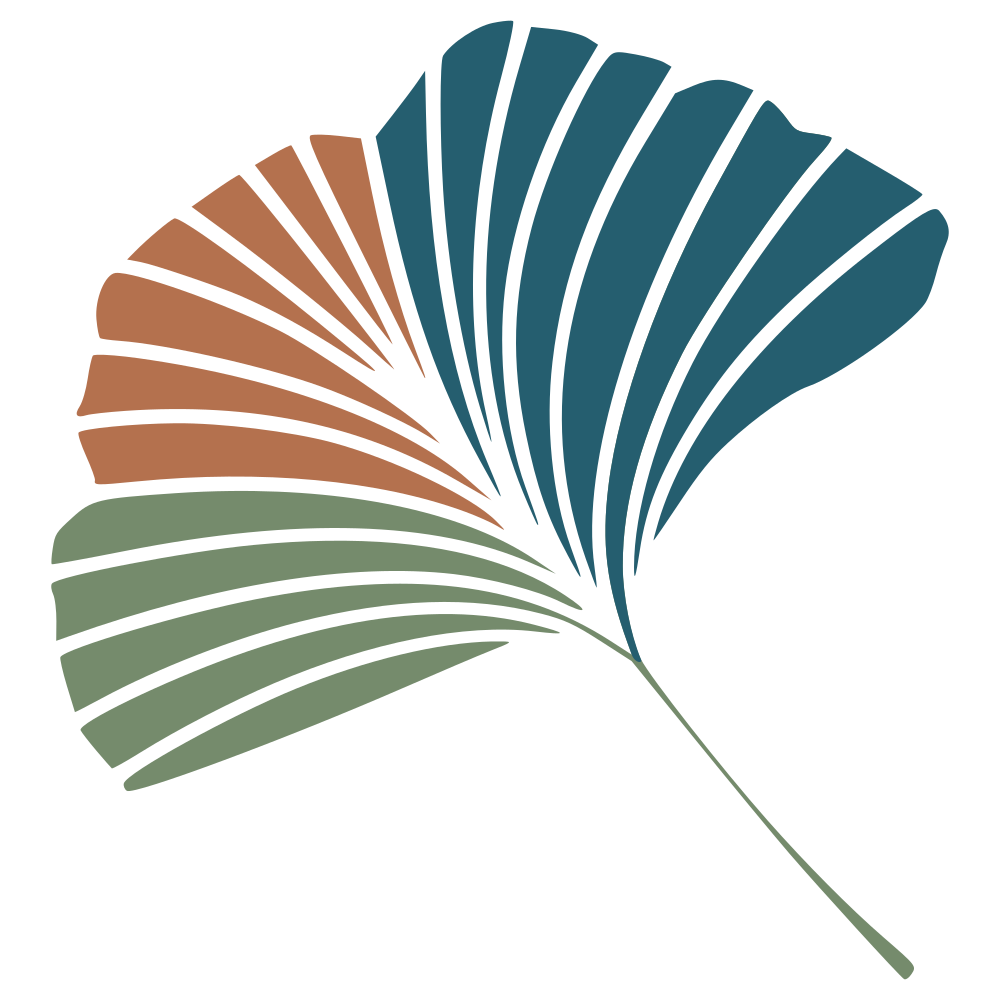using Zygote.jl

The core idea of adaptative dynamics is that if you can express the dynamics of a population according to a value of its trait, you can make a prediction about the the change in trait value, and draw inference about replacement, coexistence, and so on and so forth.

The issue with this technique (besides the simplifications, which have been discussed ad nauseam including a full issue of J. Evol. Biol.) is that it requires to do a little bit of differentiation.

In this entry, I will show how to use Zygote.jl to simplify the visualisation of some of the usual plot, i.e. the Pairwise Invasibility Plot. All the examples come from the “Hitchicker’s Guide to Adaptive Dynamics”, which is a great introduction to the core concepts.

In a nutshell, Zygote is a way to get the gradients from any expression in Julia; so let’s start with an example. We will assume a population dynamics model of the form:

$$n_r’(t) = n_r(t)\left(r-d n(t)\right)$$

The resident morph as a trait value $r$, which is its growth rate. We will assume that the mutant with trait $m$ follows the exact same functional form, and so its dynamic is given by

$$n_m’(t) = n_m(t)\left(m-d n(t)\right)$$

Adaptive dynamics assumes that (i) the resident morph is at its demographic equilibrium, so that

$$n_r^\star = \frac{r}{d}$$

The second assumption is that mutant will attempt to invade this equilibrium from rarity ($n_m \approx 0$), and that the effect of mutations of traits is small, so that $m \approx r$.

The first important value is the invasion fitness, which is the growth rate per capita of the mutant in the resident equilibrium.

In other words,

$$s_r(m) = \frac{n’_m(t)}{n_m{t}} = m - dn(t)$$

At this point, what we want is the selection gradient, which is to say the derivative of $s_r(m)$ with respect to the mutant trait value, and evaluate it at the $r = m$ point (i.e. the mutant is almost exactly the resident).

But rather than doing this with maths, let’s write code. We will assume that $r = 1.0$, and that $d = 0.2$. Keep in mind that we will be evaluating this selection gradient at $r = m$, so we end up with the following expression:

r = 1.0
d = 0.2
gradient(m -> m - d*(r/d), r)

(1.0,)


We do have a gradient with a value of $1$, so the trait is expected to evolve towards values that are always higher. This calls for the introduction of a cost to high growth rate:

$$n’_r(t) = n_r(t) \left(r - c(r) - dn(t)\right)$$

$$n’_m(t) = n_m(t) \left(m - c(m) - dn(t)\right)$$

We can re-write (after some maths, involving getting the equilibrium of the resident) our invasion fitness as

$$s_r(m) = m - c(m) - r + c(m)$$

Assumig that we pick $c(x) = 10^{-1} \text{exp}(x)$, we can then get the selection gradient:

c = (x) -> 0.1exp(x)
r = 1.0
d = 0.2
gradient(m -> m - c(m) - r + c(r), r)

(0.7281718171540954,)


One further assumption we need is that the resident $r$ is also at its evolutionary equilibrium - we should be able to see this from the Pairwise Invasibility Plot, which maps the sign of the selection gradient as a function of $r$ and $m$.

traits = LinRange(0.0, 4.0, 250)
pip = zeros(Float64, (length(traits), length(traits)))
for (i,r) in enumerate(traits)
for (j,m) in enumerate(traits)
pip[j,i] = m - c(m) - r + c(r)
end
end

heatmap(traits, traits, pip.>0.0, c=:Blues, leg=false, aspectratio=1, frame=:box)
xaxis!((0,4), "Resident")
yaxis!((0,4), "Mutant")In the above plot, the blue areas represent the mutant trait having higher fitness (i.e. it can invade), and the white areas represent negative fitness. The intersection, at some point between 2 and 3, is the evolutionary equilibrium. Any mutant above this value will be replaced by a mutant with a lower value, and any mutant below it will be replaced by a mutant with higher value. There is a more formal way of determining whether this will happen, and/or lead to coexistence, based on taking the gradient a second time (or just flipping and overlaying the PIP…), but this is not the point of this demo.

We can also approximate the equilibrium trait value a little bit better:

traits = LinRange(2.0, 2.5, 500)
fit = [first(gradient(m -> m - c(m) - r + c(r), t)) for t in traits]
plot(traits, fit, frame=:zerolines, c=:orange, lab="")
xaxis!((2, 2.5), "Trait value")r_star = traits[last(findmin(abs.(fit)))]

2.3026052104208414

So, what have we learned? Zygote is a very convenient (and fast!) way of looking at gradients and derivatives. It has a really neat syntax (you can literally define f and work with f' if you want), and is definitely useful if you feel like doing some simulations but do not feel like working out the solution of the gradient.